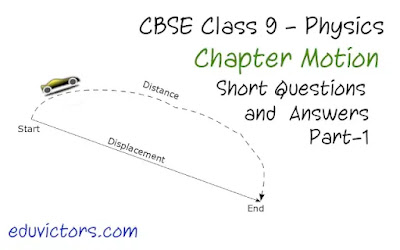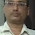## Chapter Motion: Class 9 - Physics - Short Questions and Answers - Part-1

Q1:  An object has moved through a distance. Can it have zero displacement ? If yes, support your answer with an example.

Answer: Yes, even if an object has moved through a distance, it can have zero displacement. For example an object after  moving through a certain distance (say d), comes back to its starting position. In that case, distance covered is 2d while displacement is zero.

Q2: Is displacement a scalar quantity?

Answer: No, displacement is a vector quantity because it has magnitude as well as direction.

Q3:  State whether distance is a scalar or a vector quantity.

Answer: Distance is a scalar quantity; it has magnitude but no specific direction.

Q4: Change the speed of 9 m/s into km/h.

Answer:  9m/s = 9 × 3600 / 1000 = 32.4 km/h

Q5: If a body moves with uniform velocity, what will be its acceleration?

Q6: Can the magnitude of a displacement is greater than the distance travelled by the object?

Q7: What does the odometer of an automobile measure?

Answer: The odometer of an automobile measures the distance travelled by the automobile (or vehicle).

Q8: What name is given to the speed in a specified direction?

Answer: Velocity is the name given to the speed of a body in a particular direction.

Q9: Give two examples of bodies having non-uniform motion.

Answer: Two examples of non-uniform motion:
i.  A car moving on a busy road
ii. A bee flying randomly in air.

Q10:  Name the physical quantity obtained by dividing 'Distance travelled' by 'Time taken' to travel that distance.

Answer: Speed is obtained by dividing 'distance travelled' by 'time taken' to travel that distance.

Q11: A Mango tree is standing at the corner of the park.
Ramesh says, "Since Mango tree does not move, it is at rest."
Suresh says, "Since the earth is moving, the tree is also moving."
Who is making a correct statement? What do you infer?

Answer: Both the statements are correct. Motion and rest are relative terms.

Q12: What do the following measure in a car?
a Speedometer
b Odometer

a. Speedometer of a car is used to measure the instantaneous speed of the car.
b. Odometer of a car is used to record and measure the overall distance travelled by the car.

Q13: When do the distance and magnitude of displacement become equal?

Answer: Distance and magnitude of displacement become equal when an object is tavelling in a straight path that is rectilinear path in the same direction.

Q14: Are distance and displacement time independent or time dependent quantities?

Answer: Generally the distance covered and displacement are time dependent quantities.

Q15: Give an example of a physical quantity which is time independent.

Q16: Name the physical quantity which gives us an idea of how slow or fast a body is moving.

Answer: Speed is the physical quantity that gives the idea of how slow or fast a body is moving.

Q17:     When is a body said to have uniform velocity?

Answer: When a body covers equal distances in equal intervals of time in a particular direction, it is said to have uniform velocity.

Q18: In addition to speed if we know the direction of moving object, then it is called?

Q19: Which is a vector quantity between velocity and speed?

Q20: When is a body said to have uniform velocity?

Answer: When a body covers equal distances in equal intervals of time in a particular direction, it is said to have uniform velocity.

Q21: A scooterist covers a distance of 3 kilometres in 5 minutes. Calculate his speed in metres per second (m/s).

Answer: Distance travelled = 3 km = 3000m
Time = 5 min = 5  × 60 = 300s

Speed = Distance / time = 3000 / 300 = 10 m/s

Q22: If an object covers s₁ distance in t₁ time and then covers s₂ distance in t₂ time. What is its average speed?

```   Total distance  s₁ + s₂
Avg Speed = ---------------- = ----------
Total Time  t₁ + t₂

```

Q23: Can speed be negative?

Q24: Can velocity is negative?

Q25: Under which condition is the magnitude of average velocity equal to average speed?

Answer: The magnitude of average velocity is equal to average speed when an object covers equal distances in equal intervals of time in a particular direction

Ch 8 - Motion (Q &A)
Ch8 - Motion (Worksheet)
Ch8 - Motion (MCQs)
Ch 8 - Motion (Assignment)#### 1 comment:

1.interesting